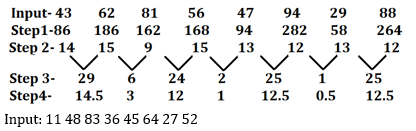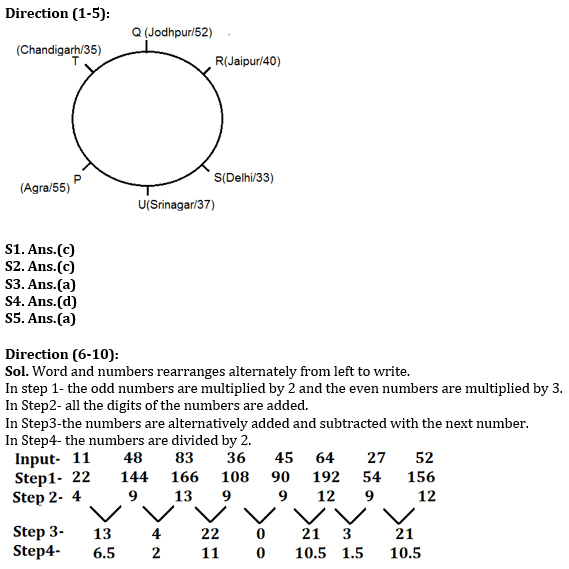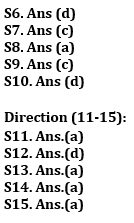Latest Banking jobs   »   Reasoning Ability Quiz For RBI Assistant/...

# Reasoning Ability Quiz For RBI Assistant/ ESIC UDC Mains 2022- 17th April

Directions (1-5): Study the following information carefully to answer the given questions.
Six persons P, Q, R, S, T and U lives in six different cities viz. Jaipur, Delhi, Agra, Chandigarh, Srinagar, and Jodhpur but not necessarily in the same order. They all came to Gurugram for a meeting and are now sitting around a circular table facing the center.
All of them are of different ages. P sits to the immediate left of the person who sits opposite to the person who lives in Jodhpur. The person who lives in Jodhpur is not an immediate neighbour of U. The youngest person is thirty-three years old. R is five years older than the second youngest person. Q who is the second oldest person does not live in Chandigarh. Q who is not an immediate neighbour of S and sits second to the left of the person who is fifty-five years old. The one who lives in Chandigarh sits opposite to S. The one who lives in Jaipur is not the oldest. T is not the youngest. R who is the fourth youngest sits second to the right of the person who lives in Srinagar. The fourth oldest person is thirty-seven years old. R sits to the immediate left of the person lives in Jodhpur. R is only three years older than fourth oldest person. The one who lives in Delhi sits second to the left of the person who is fifty-two years old. T is younger than U. U is not the oldest person.

Q1. Who amongst the following persons lives in Jaipur?
(a) S
(b) T
(c) R
(d) P
(e) Q

Q2. What is the sum of the ages of P and the youngest person?
(a) 107
(b) 92
(c) 88
(d) 72
(e) 73

Q3. How many persons are older than the person who is from Jodhpur?
(a) One
(b) Two
(c) Three
(d) Four
(e) None

Q4. Who among the following sits opposite to the person from Agra?
(a) U
(b) S
(c) T
(d) R
(e) None of these

Q5. Who among the following sits fourth to the right of S?
(a) P
(b) Q
(c) R
(d) S
(e) T

Directions (6-10): A number arrangement machine when given an input line of numbers, rearranges them following a particular rule in each step. The following is an illustration of input and rearrangement.Q6. Which of the following will be Step III for the above input?
(a) 13 4 22 0 21 3 22
(b) 13 4 22 0 22 3 21
(c) 13 4 22 1 21 3 21
(d) 13 4 22 0 21 3 21
(e) None of these

Q7. Which of the following is 2nd from the left end in last step?
(a) 1.5
(b) 0
(c) 2
(d) 6.5
(e) None of these

Q8. What is the sum of the numbers which is 3rd from the right in step 1 and 2nd from the left in last step?
(a) 194
(b) 34
(c) 267
(d) 65
(e) None of these

Q9. What is the difference between the numbers which is 3rd from the right in step 3 and 2nd from the left in step2?
(a) 9
(b) 4
(c) 12
(d) 6
(e) None of these

Q10.How many numbers are exactly divisible by 3 in step 1?
(a) Two
(b) Four
(c) Five
(d) Six
(e) None of these

Directions (11-15): In the following questions, the symbols @, #, %, \$ and * are used with the following meaning as illustrated below.
‘A @ B’ means ‘A is not smaller than B’
‘A # B’ means ‘A is neither smaller than nor equal to B’
‘A % B’ means ‘A is neither smaller than nor greater than B’
‘A \$ B’ means ‘A is not greater than B’
‘A * B’ means ‘A is neither greater than nor equal to B’

Q11. Statements:
X%W, V\$U, W#U, Z@Y, Y#X
Conclusion:
(i) V*Z
(ii) X%U
(a) If only conclusion (i) is true
(b) If only conclusion (ii) is true
(c) If either conclusion (i) or (ii) is true
(d) If neither conclusion (i) nor (ii) is true
(e) If both conclusions (i) and (ii) are true

Q12. Statements:
I%H, K\$J, G*F, J#I, H@G
Conclusion:
(i) K#I
(ii) I*F
(a) If only conclusion (i) is true
(b) If only conclusion (ii) is true
(c) If either conclusion (i) or (ii) is true
(d) If neither conclusion (i) nor (ii) is true
(e) If both conclusions (i) and (ii) are true

Q13. Statements:
V\$U, Z@Y, Y#X, W#U, X%W
Conclusion:
(i) W*Z
(ii) Z%W
(a) If only conclusion (i) is true
(b) If only conclusion (ii) is true
(c) If either conclusion (i) or (ii) is true
(d) If neither conclusion (i) nor (ii) is true
(e) If both conclusions (i) and (ii) are true

Q14. Statements:
E\$D, B#A, C*B, F\$E, D%C
Conclusion:
(i) C@F
(ii) F\$A
(a) If only conclusion (i) is true
(b) If only conclusion (ii) is true
(c) If either conclusion (i) or (ii) is true
(d) If neither conclusion (i) nor (ii) is true
(e) If both conclusions (i) and (ii) are true

Q15. Statements:
G*F, J#I, H@G, I%H, K\$J
Conclusion:
(i) G*J
(ii) K@F
(a) If only conclusion (i) is true
(b) If only conclusion (ii) is true
(c) If either conclusion (i) or (ii) is true
(d) If neither conclusion (i) nor (ii) is true
(e) If both conclusions (i) and (ii) are true

Solutions#### Congratulations!Download Hindu Review of October 2021: Free PDF